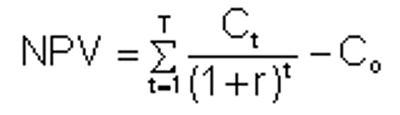#### Net Present Value (NPV)

Net Present Value (NPV) is the present value of expected cash inflows associated with an investment/project. NPV compares the value of today’s dollar to the value of that same dollar in the future, taking inflation and required returns into account. NPV is the present value of the cash flows less the initial outlay at time 0.

Ct = expected net cash flow at time t

N = estimated life of the investment

r = discount rate

Example:

Calculate the NPV of an investment project with an initial cost of \$65,000, positive cash flows of \$25,000 at the end of year 1, \$50,000 at the end of year 2, and \$75,000 at the end of year 3. Use a 15% discount rate.

NPV = -\$65,000 + \$25,000 + \$50,000+ \$75,000 = \$43,860

(1+.15)1 (1+.15)2 (1+.15)3

Since this has a positive NPV, the project should be accepted.

#### Internal Rate of Return (IRR)

Internal Rate of Return (IRR) is the rate of return that equates the PV of an investment’s benefits (positive cash flows) with the PV of its costs (negative cash flows). IRR makes the net present value of all cash flows from a particular project equal to zero (IRR = NPV = 0).

It is the “r” in the NPV formula.

Generally speaking, the higher a project's internal rate of return, the more desirable it is to undertake the project.

As such, IRR can be used to rank several prospective projects a firm is considering (note that the size of the project is similar).

0 = C0 + C1 + C2 + …. + C3

(1+IRR) (1+IRR)2 (1+IRR)3

No formula will manually solve the IRR besides a trial and error basis. However, a calculator or a computer will do it easily.

### NPV Decision Rule

· Accept projects/investments with a positive NPV – it will increase shareholder wealth· Reject projects with negative NPV – it will decrease shareholder wealth

### IRR Decision Rule

· Accept projects with an IRR that its greater than the firm/investor’s required rate of return (aka hurdle rate)· Reject projects with an IRR that is less than the firm/investor’s required rate of return (aka hurdle rate)